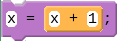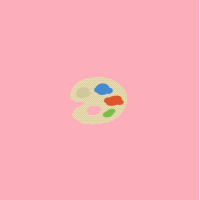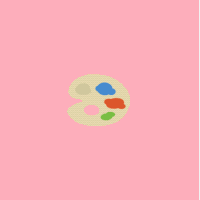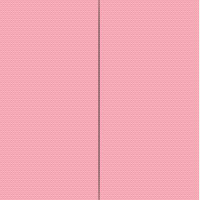# The Counter Pattern

Increasing or decreasing numbers is a common and incredibly useful pattern in programming. This "Counter Pattern" can be used to make an image fly across the screen, to count down a timer, or to keep track of clicks. Used with a variable `x` to count up by one, the Counter Pattern can look like this:Every time this code is run, it will take the current value of x, add 1, and save that as the new value of x. While this particular instance of the Counter Pattern uses addition, you could also use subtraction to count down.

## Movement with the Counter PatternThe above code uses the counter pattern in the draw loop to move three sprites. Notice that each of the three sprites moves differently depending on whether you update the sprite's `x`, `y`, or both.

## Other Uses for the Counter Pattern

Any block that takes a number as input could be animated using the counter pattern in the draw loop. Take a look at the three following examples, each of which uses the counter pattern to animate a different aspect of the image. Below each image is an example of the code that was used inside the Draw Loop to produce the animation.```sprite.rotation = sprite.rotation - 1;
``````sprite.scale = sprite.scale + 0.1;
``````width = width + 1;
height = height - 1;
ellipse(200, 200, width, height);
```

Found a bug in the documentation? Let us know at documentation@code.org